# 超声成像检测系统声波信号激励与处理技术Acoustic Signal Excitation and Processing Technology in Ultrasonic Imaging Detection System

DOI: 10.12677/JSTA.2020.81001, PDF, HTML, XML, 下载: 361  浏览: 975  科研立项经费支持

Abstract: In a process tomography system, the accuracy of projection data directly determines the imaging resolution. MAX038 chip with high working frequency is used as the signal generator in the ultra-sonic transmitting circuit, according to the sound field requirements of imaging detection. At the front-end of receiving circuit, a passive RC band-pass filter and a voltage follower with high input resistance integrated op-amp were used. The results show that the transmitted ultrasonic wave amplitude and power are stable; electromagnetic interference in the received signal is effectively eliminated, and piezoelectric charge leakage at the front-end of the receiving circuit is avoided.

1. 引言

2. 超声波激励电路的设计

2.1. 脉冲发生器电路设计

MAX038芯片输出信号幅频精度高、波形易于变换，外加少许外围电路就能够方便地输出方波、矩形波、正弦波、三角波或锯齿波。当频率调节引脚FADJ接地时，MAX038输出信号的频率 ${ƒ}_{o}$ 与电流IIN、COSC引脚外接电容Cf之间的关系为

${ƒ}_{o}=2.5V/\left[\left({R}_{W11}+{R}_{12}\right){C}_{f}\right]$ (1)

2.2. 功率放大电路设计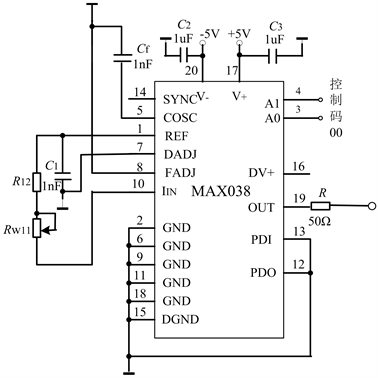Figure 1. Oscillation circuit for rectangular wave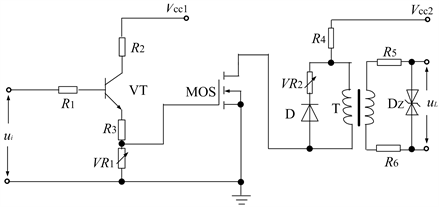Figure 2. Power amplifier circuit

${U}_{1}/{U}_{2}={N}_{1}/{N}_{2}=1/2$ (2)

${N}_{1}=\frac{{U}_{1}}{4fA×{B}_{m}}$ (3)

${Z}_{1}={\left({N}_{1}/{N}_{2}\right)}^{2}R$ (4)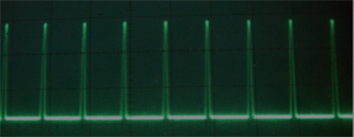Figure 3. Excitation voltage waveform

3. 信号接收电路的设计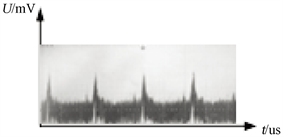Figure 4. Received signal waveform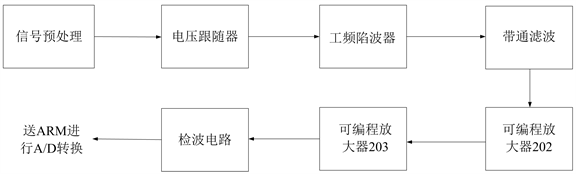Figure 5. Diagram of signal receiving circuit

3.1. 信号预处理电路设计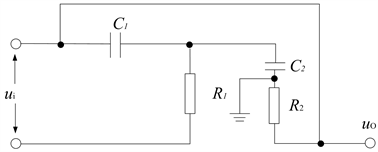Figure 6. RC band-pass filter

RC带通滤波器的传递函数(放大倍数)为输出、输入电压之比。低通滤波器的传递函数为

${\stackrel{˙}{A}}_{u}=\frac{{\stackrel{˙}{U}}_{o}}{{\stackrel{˙}{U}}_{i}}=\frac{\frac{1}{j\omega {C}_{1}}}{{R}_{1}+\frac{1}{j\omega {C}_{1}}}=\frac{1}{1+j\omega {R}_{1}{C}_{1}}$ (5)

${\stackrel{˙}{A}}_{u}=\frac{1}{1+j\frac{f}{{f}_{p}}}$ (6)

${\stackrel{˙}{A}}_{u}$ 的模为

$|{\stackrel{˙}{A}}_{u}|=\frac{1}{\sqrt{1+{\left(\frac{f}{{f}_{p}}\right)}^{2}}}$ (7)

$|{\stackrel{˙}{A}}_{u}|=\frac{\frac{f}{{{f}^{\prime }}_{p}}}{\sqrt{1+{\left(\frac{f}{{{f}^{\prime }}_{p}}\right)}^{2}}}$ (8)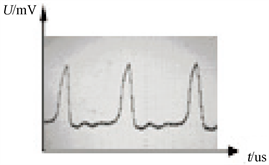Figure 7. Signal waveform after RC filtering

3.2. 电压跟随器设计

${\stackrel{˙}{A}}_{us}=\frac{{R}_{i}}{{R}_{s}+{R}_{i}}{\stackrel{˙}{A}}_{u}$ (9)

3.3. 工频陷波器设计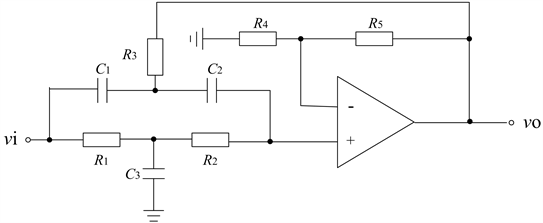Figure 8. Double T power frequency trap

$A=1+\frac{{R}_{5}}{{R}_{4}}$ (10)

3.4. 有源带通滤波器设计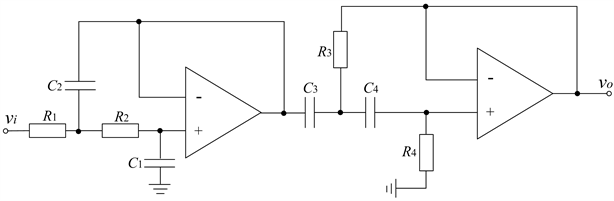Figure 9. Active band-pass filter circuit

3.5. 程控放大电路设计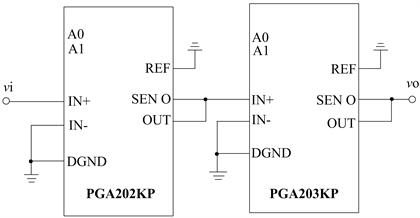Figure 10. Programmable cascade amplifier circuit

PGA放大电路是信号处理电路中唯有的数字部分，为避免外部元件引起增益、CMR等方面的误差，在电路板设计时采用了“一点接地法”。

3.6. 检波电路设计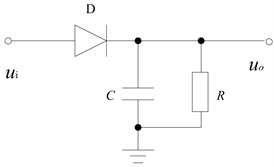Figure 11. Detection circuit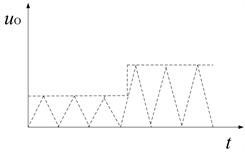Figure 12. Voltage waveform of detection circuit

4. 超声成像投影数据测量测量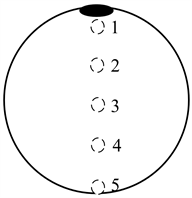Figure 13. Schematic diagram of projection data measuring device

5. 结论

NOTES

*通讯作者。

  马平, 周晓宁, 田沛. 过程层析成像技术的发展及应用[J]. 化工自动化及仪表, 2009, 36(1): 1-5.  杜运成. 基于电容层析成像技术的气液两相流特性分析[D]. 天津: 天津大学, 2011.  李楠, 徐昆, 刘逵. 基于超声层析成像技术的气/液两相流检测仿真[J]. 北京工业大学学报, 2018, 44(5):735-742.  杨键刚, 吴运新, 龚海, 李伟, 韩雷. 基于LabVIEW的电磁超声热态金属在线缺陷检测系统[J]. 传感技术学报, 2018, 31(5): 809-814.  陈文会, 丁晓鸿, 陈江宁, 刘小民. 超声波测距信号小波阈值去噪参数的选定方法[J]. 传感技术学报, 2017, 30(3): 407-410.  刘宇舜, 程登峰, 夏令志, 李森林, 程洋. 基于单通道盲源分离算法的局部放电特高频信号去噪方法[J]. 电工技术学报, 2018, 33(23): 5625-5635.  赵彩凤, 于志, 王智勇. 高频功率脉冲变压器的设计[J]. 变压器, 2003, 40(10): 6-7.